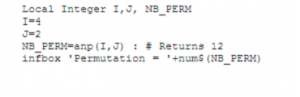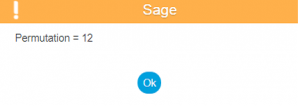# How to Calculate Permutation in Sage X3

By | September 13, 2017

Sage X3 provides a special function to calculate permutation of any integer value.

The function anp() calculates the number of permutations (for example, ordered arrangements) of p objects taken from n.

Syntax for the same is :

Syntax:

anp(N,P)

where, N and P are integer expressions. P must be in the [0,N] range.

Example:Fig. Snippet

Output:Fig. Output

Description:

The function anp(N,P) returns fac(N)/ fac(N-P).

To avoid loosing precision, the calculation algorithm does not use the factorial function. If N and P are not integer values, they are truncated.

The type of result is Integer or Decimal depending if the value is smaller or greater than 2^31-1.

Associated errors:

 Error Description 10 Not a numeric argument. 13 Out of range calculation. 50 Domain error.

Hope this helps!